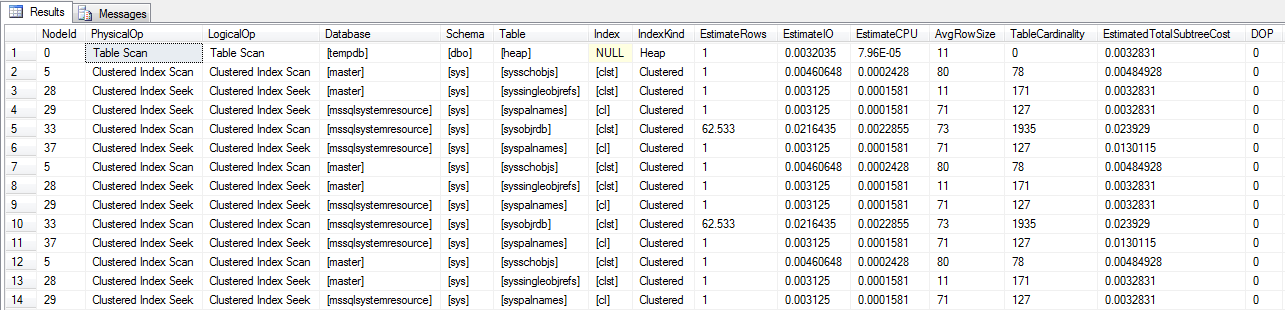# Show all index and heap access operators in the plan cache

January 27th, 2012

I recently needed a query to look into the current query plan cache and locate all actual data access operators (index scans, index seeks, table scans). This is the query I used, I decided to place it here if someone else find it useful and, more importantly, so that I can find it again when I needed it:

``````
with xmlnamespaces (default 'http://schemas.microsoft.com/sqlserver/2004/07/showplan')
select x.value(N'@NodeId',N'int') as NodeId
, x.value(N'@PhysicalOp', N'sysname') as PhysicalOp
, x.value(N'@LogicalOp', N'sysname') as LogicalOp
, ox.value(N'@Database',N'sysname') as [Database]
, ox.value(N'@Schema',N'sysname') as [Schema]
, ox.value(N'@Table',N'sysname') as [Table]
, ox.value(N'@Index',N'sysname') as [Index]
, ox.value(N'@IndexKind',N'sysname') as [IndexKind]
, x.value(N'@EstimateRows', N'float') as EstimateRows
, x.value(N'@EstimateIO', N'float') as EstimateIO
, x.value(N'@EstimateCPU', N'float') as EstimateCPU
, x.value(N'@AvgRowSize', N'float') as AvgRowSize
, x.value(N'@TableCardinality', N'float') as TableCardinality
, x.value(N'@EstimatedTotalSubtreeCost', N'float') as EstimatedTotalSubtreeCost
, x.value(N'@Parallel', N'tinyint') as DOP
, x.value(N'@EstimateRebinds', N'float') as EstimateRebinds
, x.value(N'@EstimateRewinds', N'float') as EstimateRewinds
, st.*
, pl.query_plan
from sys.dm_exec_query_stats as st
cross apply sys.dm_exec_query_plan (st.plan_handle) as pl
cross apply pl.query_plan.nodes('//RelOp[./*/Object/@Database]') as op(x)
cross apply op.x.nodes('./*/Object') as ob(ox)
``````